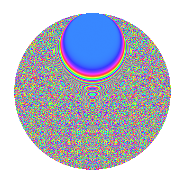# Properties

 Label 2009.2.bxLevel 2009 Weight 2 Character orbit bx Rep. character $$\chi_{2009}(19,\cdot)$$ Character field $$\Q(\zeta_{120})$$ Dimension 4352 Sturm bound 392

# Related objects

## Defining parameters

 Level: $$N$$ = $$2009 = 7^{2} \cdot 41$$ Weight: $$k$$ = $$2$$ Character orbit: $$[\chi]$$ = 2009.bx (of order $$120$$ and degree $$32$$) Character conductor: $$\operatorname{cond}(\chi)$$ = $$287$$ Character field: $$\Q(\zeta_{120})$$ Sturm bound: $$392$$

## Dimensions

The following table gives the dimensions of various subspaces of $$M_{2}(2009, [\chi])$$.

Total New Old
Modular forms 6528 4608 1920
Cusp forms 6016 4352 1664
Eisenstein series 512 256 256

## Trace form

 $$4352q + 16q^{2} + 48q^{3} + 20q^{4} + 48q^{5} - 144q^{8} + 24q^{9} + O(q^{10})$$ $$4352q + 16q^{2} + 48q^{3} + 20q^{4} + 48q^{5} - 144q^{8} + 24q^{9} + 36q^{10} + 16q^{11} + 48q^{12} - 104q^{15} - 484q^{16} + 84q^{17} + 12q^{18} + 72q^{19} - 240q^{22} + 20q^{23} + 20q^{25} + 24q^{26} - 80q^{29} + 60q^{31} + 60q^{32} + 108q^{33} - 192q^{36} - 24q^{37} + 132q^{38} + 16q^{39} - 224q^{43} - 16q^{44} + 60q^{45} + 40q^{46} + 72q^{47} - 280q^{50} - 24q^{51} + 72q^{52} - 24q^{53} - 120q^{54} - 80q^{57} - 60q^{58} + 36q^{59} - 24q^{60} + 48q^{61} - 160q^{64} + 92q^{65} + 60q^{66} + 8q^{67} - 324q^{68} - 112q^{71} + 20q^{72} + 12q^{73} + 12q^{74} - 252q^{75} - 224q^{78} + 20q^{79} - 60q^{80} - 528q^{82} + 240q^{85} + 20q^{86} - 84q^{87} - 12q^{88} - 144q^{89} + 64q^{92} - 48q^{93} + 156q^{94} + 16q^{95} - 528q^{96} - 16q^{99} + O(q^{100})$$

## Decomposition of $$S_{2}^{\mathrm{new}}(2009, [\chi])$$ into irreducible Hecke orbits

The newforms in this space have not yet been added to the LMFDB.

## Decomposition of $$S_{2}^{\mathrm{old}}(2009, [\chi])$$ into lower level spaces

$$S_{2}^{\mathrm{old}}(2009, [\chi]) \cong$$ $$S_{2}^{\mathrm{new}}(287, [\chi])$$$$^{\oplus 2}$$